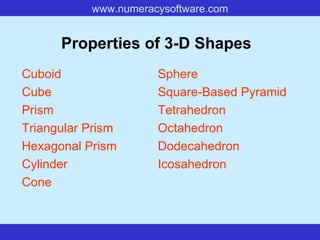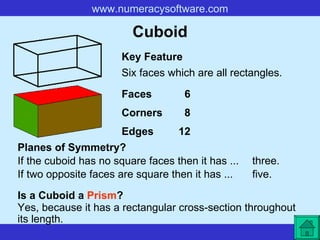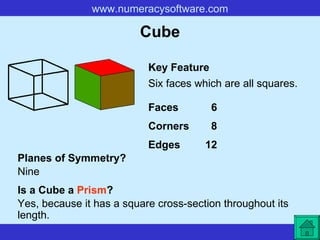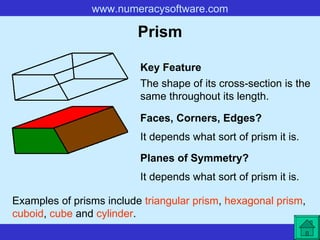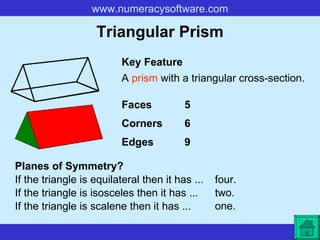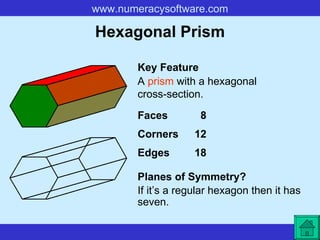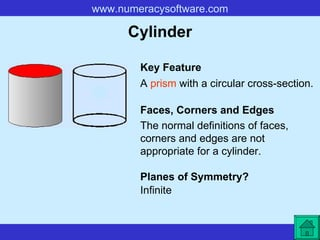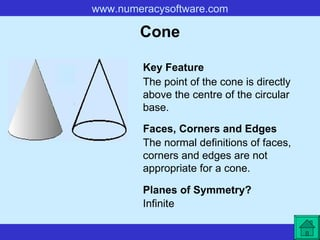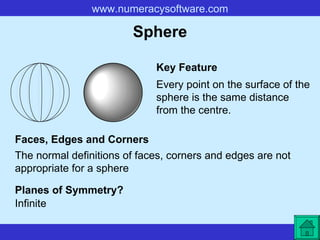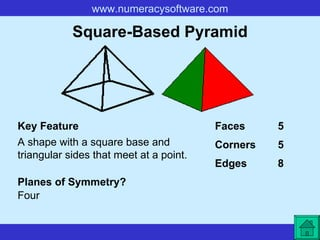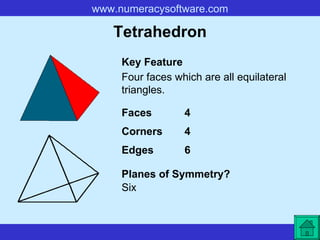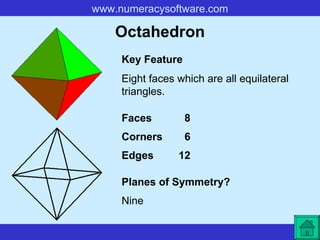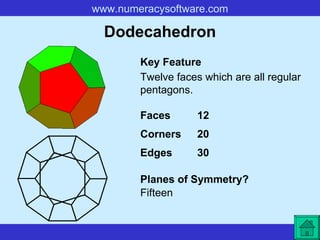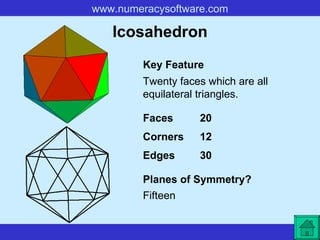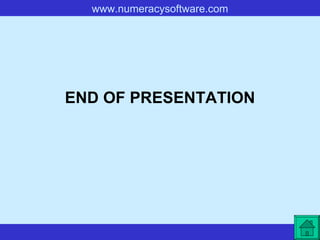1 de 153D shapesMark SantosSolid Shapesaazz0015Introduction 3D shapesInne Milanisti SihasaleProperties of 3 d shapesjennifernichols8### 3 d shapes

1. Properties of 3-D Shapes Cuboid Cube Prism Triangular Prism Hexagonal Prism Cylinder Cone Sphere Square-Based Pyramid Tetrahedron Octahedron Dodecahedron Icosahedron
2. Cuboid Six faces which are all rectangles. Key Feature Planes of Symmetry? If the cuboid has no square faces then it has ... Faces 6 Corners 8 Edges 12 Is a Cuboid a Prism ? Yes, because it has a rectangular cross-section throughout its length. If two opposite faces are square then it has ... three. five.
3. Cube Six faces which are all squares. Key Feature Planes of Symmetry? Nine Faces 6 Corners 8 Edges 12 Is a Cube a Prism ? Yes, because it has a square cross-section throughout its length.
4. Prism The shape of its cross-section is the same throughout its length. Key Feature Planes of Symmetry? It depends what sort of prism it is. Faces, Corners, Edges? It depends what sort of prism it is. Examples of prisms include triangular prism , hexagonal prism , cuboid , cube and cylinder .
5. Triangular Prism A prism with a triangular cross-section. Key Feature Planes of Symmetry? If the triangle is equilateral then it has ... Faces 5 Corners 6 Edges 9 four. If the triangle is isosceles then it has ... If the triangle is scalene then it has ... two. one.
6. Hexagonal Prism A prism with a hexagonal cross-section. Key Feature Planes of Symmetry? If it’s a regular hexagon then it has seven. Faces 8 Corners 12 Edges 18
7. Cylinder A prism with a circular cross-section. Key Feature Planes of Symmetry? Infinite Faces, Corners and Edges The normal definitions of faces, corners and edges are not appropriate for a cylinder.
8. Cone The point of the cone is directly above the centre of the circular base. Key Feature Planes of Symmetry? Infinite Faces, Corners and Edges The normal definitions of faces, corners and edges are not appropriate for a cone.
9. Sphere Every point on the surface of the sphere is the same distance from the centre. Key Feature Planes of Symmetry? Infinite Faces, Edges and Corners The normal definitions of faces, corners and edges are not appropriate for a sphere
10. Square-Based Pyramid A shape with a square base and triangular sides that meet at a point. Key Feature Planes of Symmetry? Four Faces 5 Corners 5 Edges 8
11. Tetrahedron Four faces which are all equilateral triangles. Key Feature Planes of Symmetry? Six Faces 4 Corners 4 Edges 6
12. Octahedron Eight faces which are all equilateral triangles. Key Feature Planes of Symmetry? Nine Faces 8 Corners 6 Edges 12
13. Dodecahedron Twelve faces which are all regular pentagons. Key Feature Planes of Symmetry? Fifteen Faces 12 Corners 20 Edges 30
14. Icosahedron Twenty faces which are all equilateral triangles. Key Feature Planes of Symmetry? Fifteen Faces 20 Corners 12 Edges 30
15. END OF PRESENTATION